Q-Chem 4.3 User’s Manual

# 5.4 Local MP2 Methods

## 5.4.1 Local Triatomics in Molecules (TRIM) Model

The development of what may be called “fast methods” for evaluating electron correlation is a problem of both fundamental and practical importance, because of the unphysical increases in computational complexity with molecular size which afflict “exact” implementations of electron correlation methods. Ideally, the development of fast methods for treating electron correlation should not impact either model errors or numerical errors associated with the original electron correlation models. Unfortunately this is not possible at present, as may be appreciated from the following rough argument. Spatial locality  is what permits re-formulations of electronic structure methods that yield the same answer as traditional methods, but faster. The one-particle density matrix decays exponentially with a rate that relates to the HOMO-LUMO gap in periodic systems. When length scales longer than this characteristic decay length are examined, sparsity will emerge in both the one-particle density matrix and also pair correlation amplitudes expressed in terms of localized functions. Very roughly, such a length scale is about 5 to 10 atoms in a line, for good insulators such as alkanes. Hence sparsity emerges beyond this number of atoms in 1-D, beyond this number of atoms squared in 2-D, and this number of atoms cubed in 3-D. Thus for three-dimensional systems, locality only begins to emerge for systems of between hundreds and thousands of atoms.

If we wish to accelerate calculations on systems below this size regime, we must therefore introduce additional errors into the calculation, either as numerical noise through looser tolerances, or by modifying the theoretical model, or perhaps both. Q-Chem’s approach to local electron correlation is based on modifying the theoretical models describing correlation with an additional well-defined local approximation. We do not attempt to accelerate the calculations by introducing more numerical error because of the difficulties of controlling the error as a function of molecule size, and the difficulty of achieving reproducible significant results. From this perspective, local correlation becomes an integral part of specifying the electron correlation treatment. This means that the considerations necessary for a correlation treatment to qualify as a well-defined theoretical model chemistry apply equally to local correlation modeling. The local approximations should be

• Size-consistent: meaning that the energy of a super-system of two non-interacting molecules should be the sum of the energy obtained from individual calculations on each molecule.

• Uniquely defined: Require no input beyond nuclei, electrons, and an atomic orbital basis set. In other words, the model should be uniquely specified without customization for each molecule.

• Yield continuous potential energy surfaces: The model approximations should be smooth, and not yield energies that exhibit jumps as nuclear geometries are varied.

To ensure that these model chemistry criteria are met, Q-Chem’s local MP2 methods [236, 237] express the double substitutions (i.e., the pair correlations) in a redundant basis of atom-labeled functions. The advantage of doing this is that local models satisfying model chemistry criteria can be defined by performing an atomic truncation  of the double substitutions. A general substitution in this representation will then involve the replacement of occupied functions associated with two given atoms by empty (or virtual) functions on two other atoms, coupling together four different atoms. We can force one occupied to virtual substitution (of the two that comprise a double substitution) to occur only between functions on the same atom, so that only three different atoms are involved in the double substitution. This defines the triatomics in molecules  (TRIM) local model for double substitutions. The TRIM model offers the potential for reducing the computational requirements of exact MP2 theory by a factor proportional to the number of atoms. We could also force each occupied to virtual substitution to be on a given atom, thereby defining a more drastic diatomics in molecules  (DIM) local correlation model.

The simplest atom-centered basis that is capable of spanning the occupied space is a minimal basis  of core and valence atomic orbitals on each atom. Such a basis is necessarily redundant because it also contains sufficient flexibility to describe the empty valence anti-bonding orbitals necessary to correctly account for non-dynamical electron correlation effects such as bond-breaking. This redundancy is actually important for the success of the atomic truncations because occupied functions on adjacent atoms to some extent describe the same part of the occupied space. The minimal functions we use to span the occupied space are obtained at the end of a large basis set calculation, and are called extracted polarized atomic orbitals  (EPAOs) . We discuss them briefly below. It is even possible to explicitly perform an SCF calculation in terms of a molecule-optimized minimal basis of polarized atomic orbitals  (PAOs) (see Chapter 4). To span the virtual space, we use the full set of atomic orbitals, appropriately projected into the virtual space.

We summarize the situation. The number of functions spanning the occupied subspace will be the minimal basis set dimension,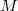, which is greater than the number of occupied orbitals,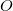, by a factor of up to about two. The virtual space is spanned by the set of projected atomic orbitals whose number is the atomic orbital basis set size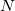, which is fractionally greater than the number of virtuals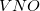. The number of double substitutions in such a redundant representation will be typically three to five times larger than the usual total. This will be more than compensated by reducing the number of retained substitutions by a factor of the number of atoms,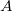, in the local triatomics in molecules model, or a factor of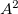in the diatomics in molecules model.

The local MP2 energy in the TRIM and DIM models are given by the following expressions, which can be compared against the full MP2 expression given earlier in Eq. (5.13). First, for the DIM model: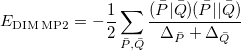(5.17)

The sums run over the linear number of atomic single excitations after they have been canonicalized. Each term in the denominator is thus an energy difference between occupied and virtual levels in this local basis. Similarly, the TRIM model corresponds to the following local MP2 energy: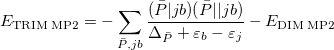(5.18)

where the sum is now mixed between atomic substitutions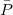, and nonlocal occupied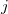to virtualsubstitutions. See Refs. Lee:2000b,Head-Gordon:1999c for a full derivation and discussion.

The accuracy of the local TRIM and DIM models has been tested in a series of calculations [236, 237]. In particular, the TRIM model has been shown to be quite faithful to full MP2 theory via the following tests:

• The TRIM model recovers around 99.7% of the MP2 correlation energy for covalent bonding. This is significantly higher than the roughly 98–99% correlation energy recovery typically exhibited by the Saebo-Pulay local correlation method . The DIM model recovers around 95% of the correlation energy.

• The performance of the TRIM model for relative energies is very robust, as shown in Ref. Lee:2000b for the challenging case of torsional barriers in conjugated molecules. The RMS error in these relative energies is only 0.031 kcal/mol, as compared to around 1 kcal/mol when electron correlation effects are completely neglected.

• For the water dimer with the aug-cc-pVTZ basis, 96% of the MP2 contribution to the binding energy is recovered with the TRIM model, as compared to 62% with the Saebo-Pulay local correlation method.

• For calculations of the MP2 contribution to the G3 and G3(MP2) energies with the larger molecules in the G3-99 database , introduction of the TRIM approximation results in an RMS error relative to full MP2 theory of only 0.3 kcal/mol, even though the absolute magnitude of these quantities is on the order of tens of kcal/mol.

## 5.4.2 EPAO Evaluation Options

When a local MP2 job (requested by the LOCAL_MP2 option for CORRELATION) is performed, the first new step after the SCF calculation is converged is to extract a minimal basis of polarized atomic orbitals (EPAOs) that spans the occupied space. There are three valid choices for this basis, controlled by the PAO_METHOD and EPAO_ITERATE keywords described below.

• Uniterated EPAOs: The initial guess EPAOs are the default for local MP2 calculations, and are defined as follows. For each atom, the covariant density matrix (SPS) is diagonalized, giving eigenvalues which are approximate natural orbital occupancies, and eigenvectors which are corresponding atomic orbitals. Theeigenvectors with largest populations are retained (whereis the minimal basis dimension for the current atom). This nonorthogonal minimal basis is symmetrically orthogonalized, and then modified as discussed in Ref. Lee:2000c to ensure that these functions rigorously span the occupied space of the full SCF calculation that has just been performed. These orbitals may be denoted as EPAO(0) to indicate that no iterations have been performed after the guess. In general, the quality of the local MP2 results obtained with this option is very similar to the EPAO option below, but it is much faster and fully robust. For the example of the torsional barrier calculations discussed above , the TRIM RMS deviations of 0.03 kcal/mol from full MP2 calculations are increased to only 0.04 kcal/mol when EPAO(0) orbitals are employed rather than EPAOs.

• EPAOs: EPAOs are defined by minimizing a localization functional as described in Ref. Lee:2000c. These functions were designed to be suitable for local MP2 calculations, and have yielded excellent results in all tests performed so far. Unfortunately the functional is difficult to converge for large molecules, at least with the algorithms that have been developed to this stage. Therefore it is not the default, but is switched on by specifying a (large) value for EPAO_ITERATE, as discussed below.

• PAO: If the SCF calculation is performed in terms of a molecule-optimized minimal basis, as described in Chapter 4, then the resulting PAO-SCF calculation can be corrected with either conventional or local MP2 for electron correlation. PAO-SCF calculations alter the SCF energy, and are therefore not the default. This can be enabled by specifying PAO_METHOD as PAO, in a job which also requests CORRELATION as LOCAL_MP2.

PAO_METHOD
 Controls the type of PAO calculations requested.

TYPE:
 STRING

DEFAULT:
 EPAO For local MP2, EPAOs are chosen by default.

OPTIONS:
 EPAO Find EPAOs by minimizing delocalization function. PAO Do SCF in a molecule-optimized minimal basis.

RECOMMENDATION:
 None

EPAO_ITERATE
 Controls iterations for EPAO calculations (see PAO_METHOD).

TYPE:
 INTEGER

DEFAULT:
 0 Use uniterated EPAOs based on atomic blocks of SPS.

OPTIONS:Optimize the EPAOs for up toiterations.

RECOMMENDATION:
 Use default. For molecules that are not too large, one can test the sensitivity of the results to the type of minimal functions by the use of optimized EPAOs in which case a value of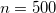is reasonable.

EPAO_WEIGHTS
 Controls algorithm and weights for EPAO calculations (see PAO_METHOD).

TYPE:
 INTEGER

DEFAULT:
 115 Standard weights, use 1and 2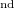order optimization

OPTIONS:
 15 Standard weights, with 1order optimization only.

RECOMMENDATION:
 Use default, unless convergence failure is encountered.

## 5.4.3 Algorithm Control and Customization

A local MP2 calculation (requested by the LOCAL_MP2 option for CORRELATION) consists of the following steps:

• After the SCF is converged, a minimal basis of EPAOs are obtained.

• The TRIM (and DIM) local MP2 energies are then evaluated (gradients are not yet available).

Details of the efficient implementation of the local MP2 method described above are reported in the recent thesis of Dr. Michael Lee . Here we simply summarize the capabilities of the program. The computational advantage associated with these local MP2 methods varies depending upon the size of molecule and the basis set. As a rough general estimate, TRIM MP2 calculations are feasible on molecule sizes about twice as large as those for which conventional MP2 calculations are feasible on a given computer, and this is their primary advantage. Our implementation is well suited for large basis set calculations. The AO basis two-electron integrals are evaluated four times. DIM MP2 calculations are performed as a by-product of TRIM MP2 but no separately optimized DIM algorithm has been implemented.

The resource requirements for local MP2 calculations are as follows:

• Memory: The memory requirement for the integral transformation does not exceed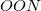, and is thresholded so that it asymptotically grows linearly with molecule size. Additional memory of approximately 32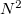is required to complete the local MP2 energy evaluation.

• Disk: The disk space requirement is only about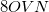, but is not governed by a threshold. This is a very large reduction from the case of a full MP2 calculation, where, in the case of four integral evaluations,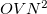/4 disk space is required. As the local MP2 disk space requirement is not adjustable, the AO2MO_DISK keyword is ignored for LOCAL_MP2 calculations.

The evaluation of the local MP2 energy does not require any further customization. An adequate amount of MEM_STATIC (80 to 160 Mb) should be specified to permit efficient AO basis two-electron integral evaluation, but all large scratch arrays are allocated from MEM_TOTAL.

## 5.4.4 Examples

Example 5.78  A relative energy evaluation using the local TRIM model for MP2 with the 6-311G** basis set. The energy difference is the internal rotation barrier in propenal, with the first geometry being planar trans, and the second the transition structure.

$molecule 0 1 C C 1 1.32095 C 2 1.47845 1 121.19 O 3 1.18974 2 123.83 1 180.00 H 1 1.07686 2 121.50 3 0.00 H 1 1.07450 2 122.09 3 180.00 H 2 1.07549 1 122.34 3 180.00 H 3 1.09486 2 115.27 4 180.00$end

$rem METHOD local_mp2 BASIS 6-311g**$end

@@@

$molecule 0 1 C C 1 1.31656 C 2 1.49838 1 123.44 O 3 1.18747 2 123.81 1 92.28 H 1 1.07631 2 122.03 3 -0.31 H 1 1.07484 2 121.43 3 180.28 H 2 1.07813 1 120.96 3 180.34 H 3 1.09387 2 115.87 4 179.07$end

$rem CORRELATION local_mp2 BASIS 6-311g**$end